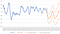# DI: Excel Forecasts a 40% Plummet of HK Housing Price!

Time series forecasting is important in financial analysis, and there are at least 3 common forecasting approaches, viz. (1) by projecting the past into the future; (2) by a forecasting model based on apriori knowledge; and (3) by regression. This article will discuss in details only about the first one.

Approach 1: By projecting the past into the future

There are at least 2 tools provided by Excel to carry out the forecast. The first tool is based on the assumption that the future time series will trace similarly to the past track based on some pre-set functions.

In other words, it is based on a curve-fitting with some pre-set functions, such as (1) Linear Function, (2) Exponential Function, (3) Polynomial Function (can choose different degrees), (4) Power Function, (5) Logarithmic Function, (6) Moving Average.

When you insert a graph and click the curve, there will be a menu shown for choosing different trendline format as shown in Figure 1. It also allows displaying of the forecasted curve, the Equation and the R-Squared. Figure 1 is an example showing a best-fit curve by a second-degree polynomial function on the time series. It shows the Forecasted Time Series, the Equation and the R-Squared on the graph.

Another forecasting tool provided by Excel is more sophisticated. It is based on moving average method, and it provides a separate forecasting sheet showing the curve, statistical results on upper confidence bound, lower confidence bound, and errors of the forecast.

Figures 2 and 3 show the forecasting sheet and the forecasted curve. It can be obtained by clicking Data, and then Forecasting Sheet on the top menu. By choosing the Timeline Range and the Value Range, and the Seasonality Period (or left it be chosen automatically), etc, it shows the forecasted time series, together with the upper and lower confidence bounds as shown.

Figure 3 is an example of the forecasted time series based on the year-on-year change of the monthly housing price index provided by the Rating and Valuation Department, from Jan 1983 to Feb 2019. Since it shows a very strong cyclical pattern, the choice of seasonality period would be critical in the forecasting. The Seasonality Period in the above example is 43 months by choosing the Detect Automatically option. You may try applying different Seasonality Period and will find a very different forecast in this example. (in case you need the data series, you may download from RVD homepage, or send me a message to request).Figure 3 A Forecasted Time Series on the YoY Housing Price Index of Hong Kong 2019–2026

Based on the Excel forecasting function and RVD data series on housing price of Hong Kong, it shows that the housing price would go down about 40% at the end of 2019! With very wide confidence bound from -60% to -10%.

However, the Excel built-in forecasting tool is based on the moving average method. There are more sophisticated forecasting methods, such as ARMA, ARIMA and the Hodrick-Prescott filter (HP Filter) which are not directly provided by Excel. You are encouraged to try different methods to test the differences, and also keep track of the accuracy of the forecasted data by comparing them with the actual outcomes in the future.

The second and third approaches would be discussed separately.

Written by